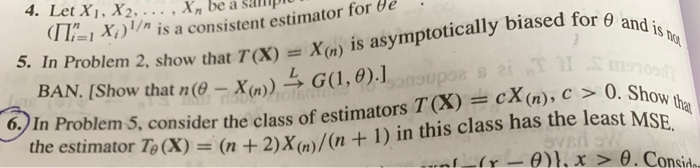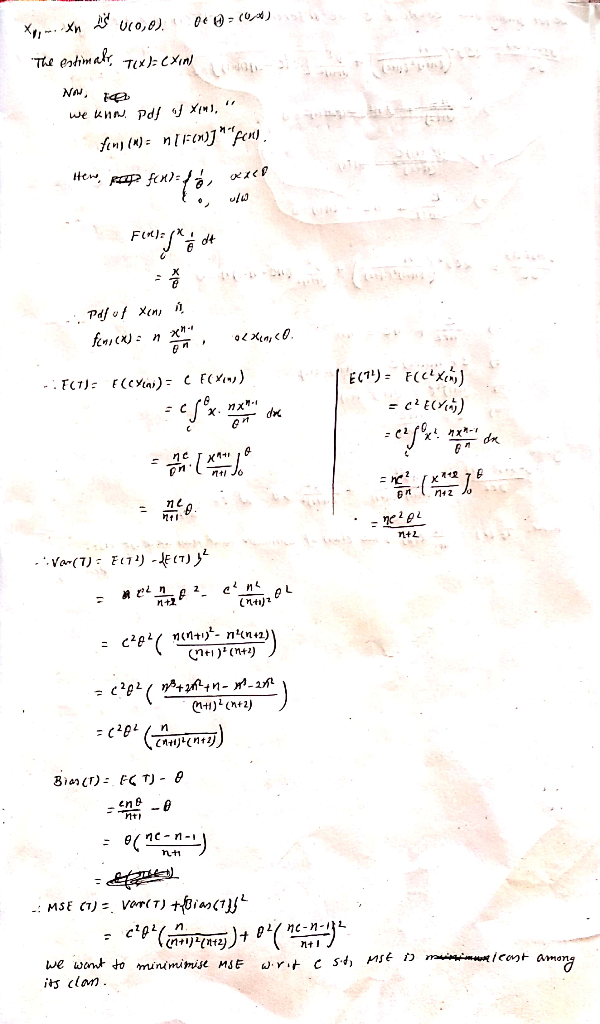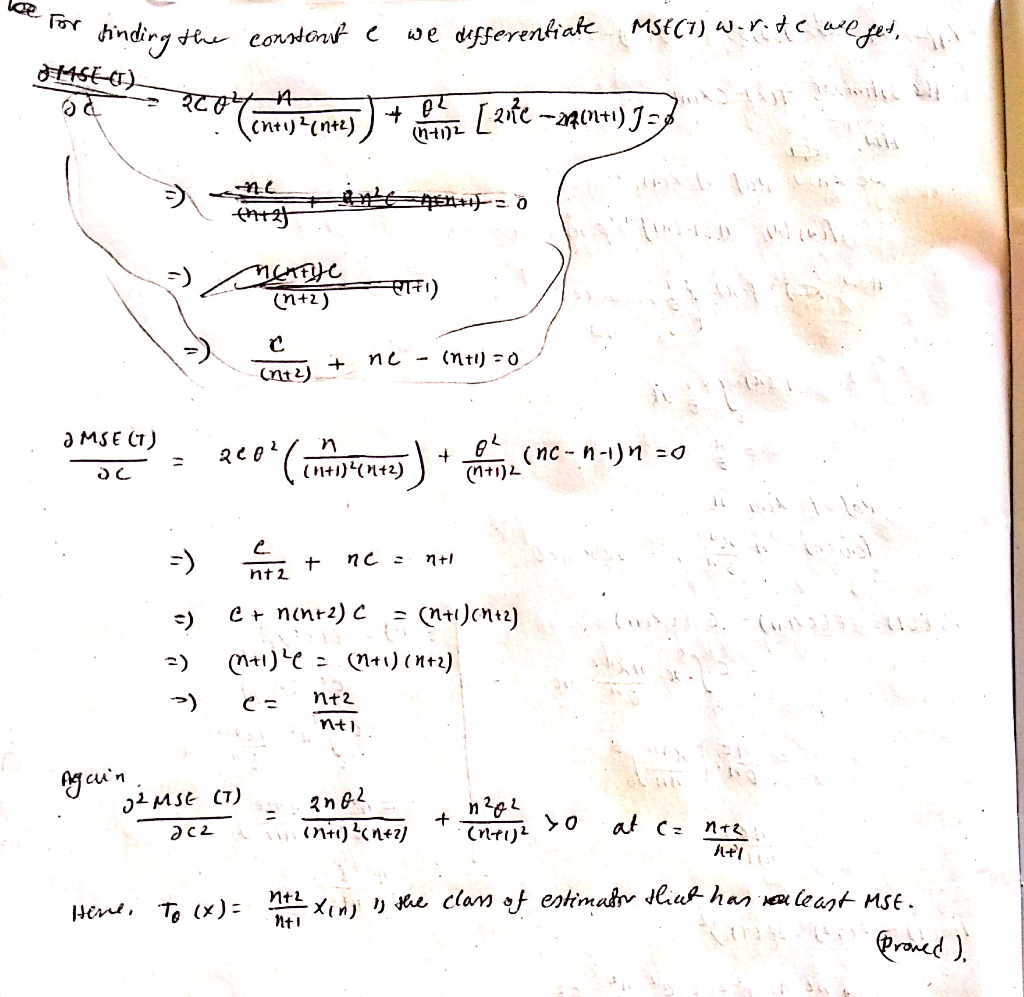# 4. Let Xi. X2. . Xnbe ap (1 I: 1 Xi ) 1/n is a consistent estimator for θ e . BAN. [Show that n(θ...4. Let Xi. X2. . Xnbe ap (1 I: 1 Xi ) 1/n is a consistent estimator for θ e . BAN. [Show that n(θ-X(n)) G (1, θ the estimator T0(X) = (n + 2)X(n)/(n + 1) in this class has the least MSE. an 5. In Problem 2, show that TX)Xm) is asymptotically biased for o 6.In Problem 5, consider the class of estimators T(X) cX(n), c 0. Sho##### Add Answer of: 4. Let Xi. X2. . Xnbe ap (1 I: 1 Xi ) 1/n is a consistent estimator for θ e . BAN. [Show that n(θ...
More Homework Help Questions Additional questions in this topic.

• #### Prove that the OLS estimator As for β in the linear regression model is consistent Let's first sh...

Need Online Homework Help?